Chocolates

In the market have 3 kinds of chocolates.

How many ways can we buy 14 chocolates?

Result

n =  120

Solution:Leave us a comment of this math problem and its solution (i.e. if it is still somewhat unclear...):Be the first to comment!To solve this verbal math problem are needed these knowledge from mathematics:

See also our combinations with repetition calculator. Would you like to compute count of combinations?

Next similar math problems:

1. ConfectioneryThe village markets have 5 kinds of sweets, one weighs 31 grams. How many different ways a customer can buy 1.519 kg sweets.
2. Virus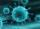We have a virus that lives one hour. Every half hour produce two child viruses. What will be the living population of the virus after 3.5 hours?
3. Fish tankA fish tank at a pet store has 8 zebra fish. In how many different ways can George choose 2 zebra fish to buy?
4. First class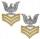The shipment contains 40 items. 36 are first grade, 4 are defective. How many ways can select 5 items, so that it is no more than one defective?
5. Candy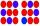How many ways can divide 10 identical candies to 5 children?
6. Cinema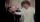How many ways can be divided 11 free tickets to the premiere of "Jáchyme throw it in the machine" between 6 pensioners?
7. TrinityHow many different triads can be selected from the group 43 students?
8. Committees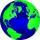How many different committees of 6 people can be formed from a class of 30 students?
9. Hockey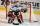Hockey match ended 8:2. How many different matches could be?
10. ChordsHow many 4-tones chords (chord = at the same time sounding different tones) is possible to play within 7 tones?
11. Hockey game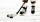In the hockey game was made 6 goals. Czech played against Finland. Czechs won 4:2. In what order to fall goals? How many game sequence was possible during the game?
12. Big factorial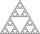How many zeros end number 116! ?
13. Circus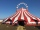On the circus performance was 150 people. Men were 10 less than women and children 50 more than adults. How many children were in the circus?
14. Calculation of CNCalculate: ?
15. One greenIn the container are 45 white and 15 balls. We randomly select 5 balls. What is the probability that it will be a maximum one green?
16. Theorem proveWe want to prove the sentence: If the natural number n is divisible by six, then n is divisible by three. From what assumption we started?
17. Sum of two primesChristian Goldbach, a mathematician, found out that every even number greater than 2 can be expressed as a sum of two prime numbers. Write or express 2018 as a sum of two prime numbers.